Divisor Drips andSquare Root Waves Prime Numbers are the Holes in Complex Composite Number Patterns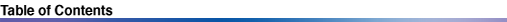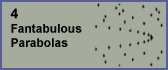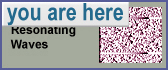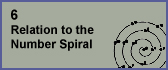5. Resonating Waves Parabolas are scattered throughout the divisor plot, not just along the square root boundary. They are most clearly-visible among the divisors of the higher numbers. They come in many varieties, and I have reason to believe that each is nestled inside of a unique local environment.Long after discovering these parabolas, I came to realize that they can be interpreted as resonating waves among divisor pairs. In this chapter, we will come to an understanding of what makes parabolas resonate so much - not only in the visual mind, but in the relationships among their divisor pairs. Let's start by looking at a special class of parabolas whose vertices lie at x which are powers of two (x = 2n). The illustration below shows a few such parabolas above and below the square root boundary in a window with the y range scaled 3 times the x range to make them easier to see. The square root boundary is indicated with a mostly-horizontal line. The left panel shows a pair of divisors located at the vertices of parabolas at x = 8192 = 213. The right panel shows a pair of divisors located at the vertices of parabolas at x = 16384 = 214, plus its square root. Mersenne primes are the primes of the form 2n-1. There are gaps in x immediately to the left of these parabola vertices (such as the Mersenne prime 213-1, indicated here). These visual gaps might help to provide some insight into the Mersenne primes.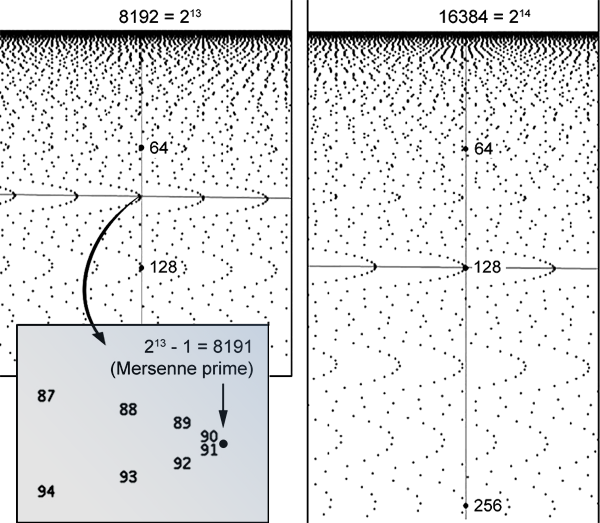Notice that the y values of these 2n numbers are themselves 2n numbers. It turns out that there are many curves in the divisor plot that contain 2n parabolas, including the square root boundary itself (they are located at x = 2n, where n is even, as we saw in the example of 214). Now take a look at this illustration: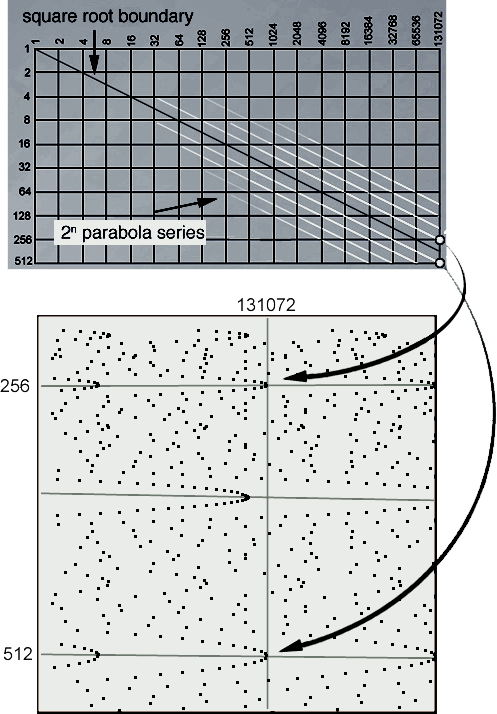This image shows a schematic of these curves in the divisor plot. Both the x and y dimensions are scaled exponentially, so that x = 2n, and y = 2k. The values of n and k are integers, represented by the grid lines in the graph. Notice that the square root boundary (the darker line cutting diagonally through the grid) appears straight as a result of this scaling. On either side of the square root boundary are white lines where 2n parabola vertices lie (specifically, at the locations where the graph lines cross). Two examples of parabola vertices are indicated by dots at the bottom-right of the graph. Notice that the white lines start off transparent and get brighter towards the lower-right. I rendered them this way because it is difficult to detect 2n parabolas at smaller x, or farther from the square root boundary. This is not to say that they don't exist - just that I was not able to identify any.If you look closely in the image above you can make out a few parabola series that lie at y values sandwiched in-between the parabola curves represented in the graph. It appears that all parabola series follow similar curves. In the image below, we see a pair of parabolas lying above and below the square root boundary. Notice how each divisor is located on the opposite arm of the parabola in relation to the other divisor in the conjugate pair. These opposing arms contain conjugate divisors. This is a property of all the non-square root parabolas, which come in pairs.
 The illustration shows how the number 10366 is the product of 71 and 146, and it is also the product of 73 and 142. Is there any significance to the fact that these pairs are so close? Notice that 10368 has a pair of divisors each lying at parabola vertices.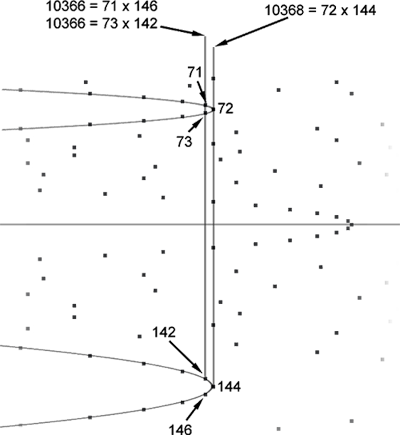In order to better understand these parabolas, let us first explore the divisors that lie exactly on the vertices. You may have noticed in the above example that 144 = 72 x 2. Below we see another example of pairs if parabolas whose vertices have the same relationship, i.e., the y value of the lower vertex is twice that of the y value of the upper vertex.In the following image we see pairs of parabolas whose vertices have a different of one forth, i.e., 90 x 4 = 360.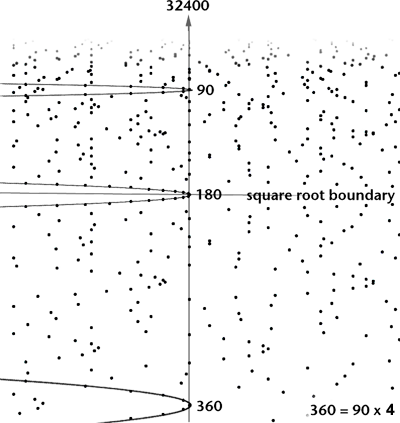We are finding parabola vertices with divisor pairs related by integer values. The illustration below shows a parabola at left, lying on the square root boundary with vertex at (4761, 69). It is represented by a square. At right is a pair of parabolas whose vertices are (4802, 49), and (4802, 98), This pair is represented by a 1x2 rectangle.Below is an illustration of a larger area showing several streams of parabolas, in the vicinity of one million. This graph has been compressed in the horizontal axis to make the parabolas easier to see. Near the middle of the graph is shown the square root of one million (1000). Just to the left of that is shown a pair of divisors (707 x 1414) for 999698. These have a ratio of 2. To the left of that is shown a pair of divisors (577 x 1731) for 998787. They have a ratio of 3. Another pair of divisors is shown (500 x 2000) for one million. These have a ratio of 4. This graph shows parabola vertices corresponding to divisor pairs with integer-number ratios.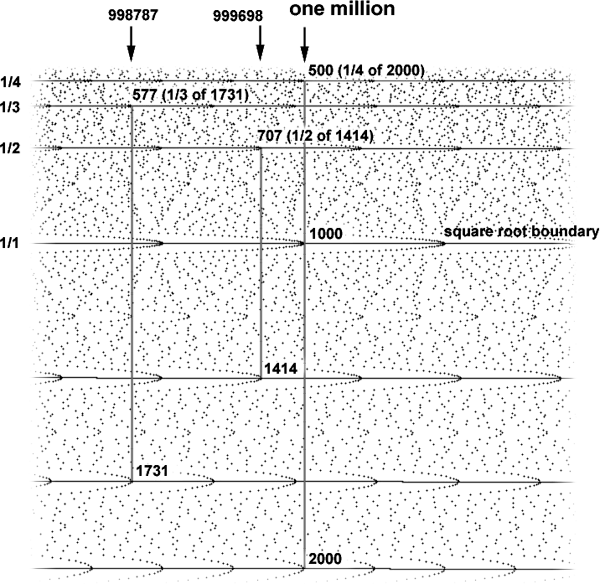With this discovery that parabola vertices are associated with divisor pairs with integer ratios, I found that I could plot a subset of divisors to view parabolas more clearly. The illustration below shows the region near the square root of one million, with only certain divisors visible, according to a threshold criterion: only divisors that come in pairs whose ratios are close to an integer value, using an arbitrary cutoff threshold chosen to maximize parabola visibility.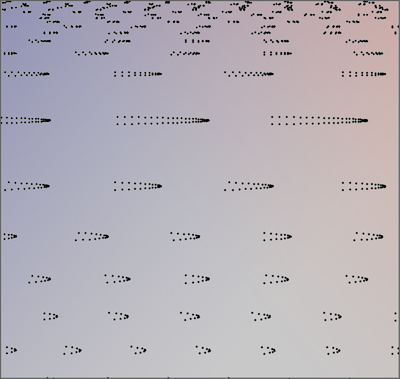Presto! We now know how to isolate parabolas in the divisor plot using math, and not just our eyes. In fact, given this knowledge, it's very easy to predict where a parabola vertex is living in the divisor plot without ever having to lay your eyes upon it. All you have to do is pick a number (say, 347), and then multiply it by some integer (small ones are easiest - say, 3). In this case you end up with 1041. Now, multiply 347 by 1041, and you get 361227. Your mystery parabola lives at x=361227, y=347. You can confirm this by visiting 361227 on the number line, and then scrolling down along the divisors until you get to your original number: 347. There you will see a lovely parabola vertex, waiting for your visit, smiling sideways at you. Parabolas and Exponentiation Considering the discovery of the square root parabolas and the 2n parabolas, I wondered if there might be some connection between exponentiation and the existence of parabolas. The illustration below makes this question visual - it shows the three usual operations of ordinal numbers: addition, multiplication, and exponentiation.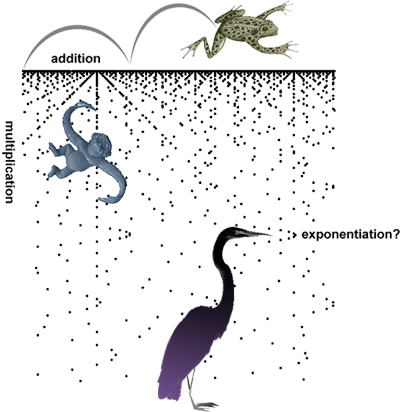I am not sure if parabolas are associated with exponentiation in general, although there is at least a strong correlation to squared numbers. Since we know that parabola vertices are associated with divisor pairs that have integer ratios, we can come up with the following bit of math: Product x is equal to y1 times y2. If y2 is twice the size of y1, we can express x as: y1 x y1 x 2 or y12 x 2 If y2 is five times the size of y1, then it can be expressed as: y1 x y1 x 5 or y12 x 5 In both cases, we end up with one squared value and a multiplier. We can generalize this to: y1 x y1 x n or y12 x n This is consistent with a discovery that Rob Sacks made while exploring parabola series. He observed that every parabola below the square root boundary can be generated by taking a square root boundary parabola and scaling all its 2D divisor locations by an integer n. Its complimentary parabola (lying above the square root boundary) can be found by dividing the y values of those divisors by n. This is illustrated below. The square root parabola P6 at left has both the x,y values of all its divisors multiplied by n = 2 to create the larger, fatter parabola at lower-right. Dividing the y values of those divisors by n generates the smaller, skinnier parabola above that.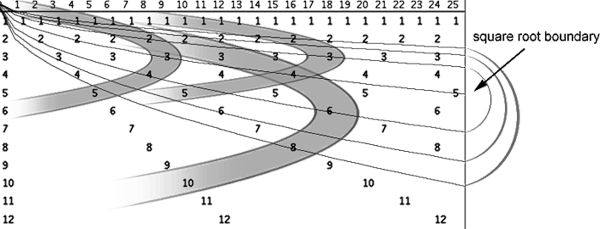All derived parabolas fall along curves above and below the square root boundary, as shown below. This scaling in y may explain why parabolas are fatter below the boundary and skinnier above the boundary. Each of these curves can be expressed as follows: y = (sqrt(x)) x n And so, the curves that complimentary parabolas lie on can be expressed as: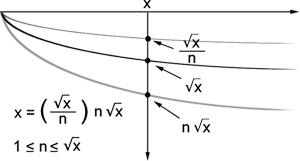Resonance I have started to think of parabolas as representing the places in the divisor plot where divisor pairs resonate with each other by whole number values. The strongest resonating wave is at the square root, where divisor pairs are close to the same (or identical in the case of the roots of perfect squares). Slightly less resonating are the divisor pairs that have a relationship near 2 to 1, as we have seen. And slightly less than that are the ones with a relationship near 3 to 1. And so-on. Perhaps thinking in terms of resonance might help us get a better understanding as to why these parabolas exist. Why Do Parabolas Point In One Direction? There is a fundamental question that David Cox raises: why are the arms of these parabolas all left-opening?  (I like to describe the parabolas as waves pushing rightward, but it doesn't matter - the question still remains: why is it not the other way around?) Cox points out that it remains to be proven that all parabolas are left-opening, and why. My suspicion is that all of them are indeed left-opening, and the reason is related to the way some divisor pairs converge towards integer ratios as you move along the number line towards larger numbers. For me, it is more productive to let my mind wander from left to right, in the direction of the pointing fingers - towards the higher numbers. The following imaginary scenario illustrates this direction of thought. Sugar Cube Packaging Imagine that you have 100 sugar cubes, and imagine that each sugar cube is 1 x 1 x 1 centimeters to a side. In order for them to fit snugly into a square tray, you need the tray to be 10 centimeters to a side. But if all you have is a 9 x 11 centimeter tray, you could put 99 sugar cubes in the tray, although you have to toss out one sugar cube. It's not a square, but pretty close, as shown at left in the following illustration.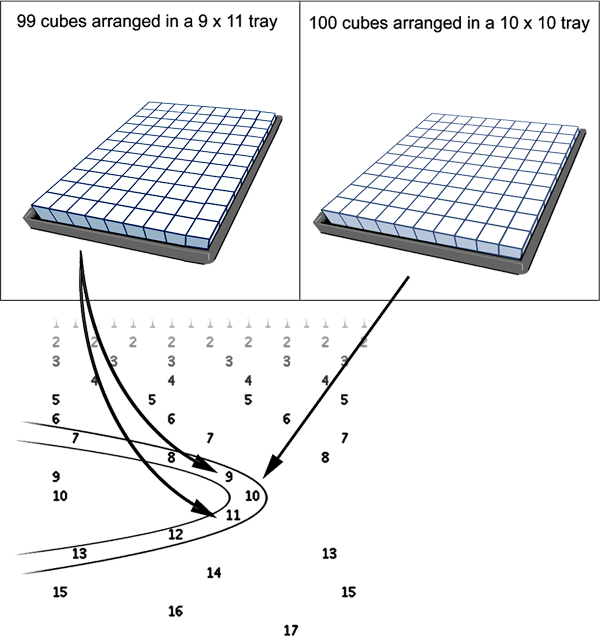In this illustration these sugar cube trays are associated with the two highest x values of parabola P20. Again we encounter the notion of squareness and almost squareness. The 9 x 11 tray of 99 sugar cubes is as close as you can get to a square tray of 100 cubes without going over 100. If you prefer square trays, it's the next best thing. Your other choice is to get an 11x11 tray, but then you would have empty space in the tray without any sugar cubes. The illustration below shows what various sugar cube trays would look like if viewed from the top, corresponding to divisors along P20. Look at the path that traverses the curve of the parabola, crossing over the square root of 100: its divisor pairs flip over and trade places at that point. This path is represented in the schematic graph at the right of the illustration.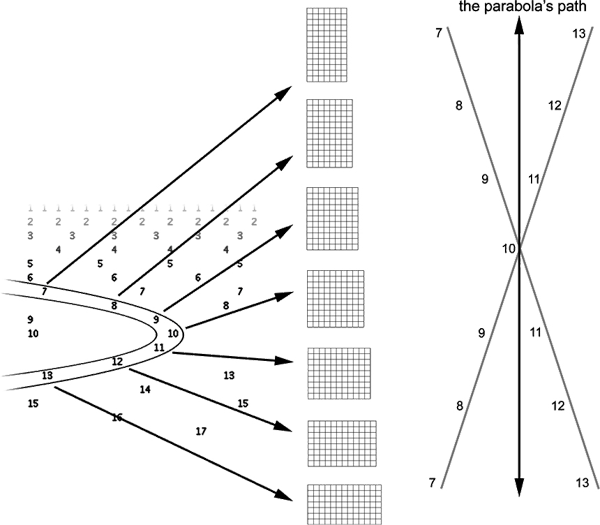Contrast the parabola's nonlinear path with the linear path of the number line, which marches from left to right. As the number line approaches 100, the divisor pairs near the square root boundary converge on the parabola's vertex. And then, suddenly: POOF. No more divisors! We have landed on the number 101 (which happens to be a prime). My question is: why do we not find any numbers that have divisors close to the square root boundary...until we get to 104? What accounts for this "squareless" gap? First Day at the Factory A little narrative might help enhance intuition. Imagine that you work at a sugar cube factory, and your job is to put the cubes into large, flat, square trays, and package them for shipping. It's your first day on the job. You have been instructed to package as many sugar cubes into a single square tray as you can. You see that there are many square (and almost square) trays, and there are many different sizes. So, you start by grabbing the biggest tray you can find, and you begin filling it with sugar cubes. The first tray you grab happens to be a 9x9 tray. You start filling it with sugar cubes, packing them tightly. Soon it gets filled up, and you look around to see if there are any bigger trays. You find one that is slightly bigger (9x10, to be exact), and then you move all the cubes into that tray, and you continue adding more cubes. Soon, that tray gets filled up, and you decide to find a bigger tray (10x10). You soon discover that this factory is well-stocked with large square trays, and if you are willing to look around, you'll find a bigger tray to put the cubes in. This process is illustrated below.This little narrative has a rhythm: For each cycle, something changes gradually (adding more cubes), and then there is a sudden change (switching to a new tray). This rhythm is echoed in the illustration of the vertices of the square root parabolas P18 through P21. As you scan from left to right along a parabola, its width tapers down until it reaches its vertex and then...poof...empty space (like the new space in the bigger tray which will get filled up with more sugar cubes). Then the cycle repeats itself. What I have just described to you is a qualitative description of both the directional and rhythmic character of the square root parabolas, rather than a mathematical explanation for why these parabolas point in the direction that they do. So, we are still left without a satisfactory answer. I'm not even sure if I have qualitatively answered the question as to why almost identical (or almost integer-ratio) divisor pairs sit to the left of parabola vertices, and not to the right. I feel as if we are close to arriving at a conjecture, but we are not there yet! Hopefully my qualitative description will provide some narrative lubrication for those of you with good math brains. Listen to the Resonating Waves Parabolas in the divisor plot are both aesthetically compelling as well as mathematically rich. Their existence raises many questions. For instance, could it be that ALL parabola series tessellate the entire domain of the divisor plot, as we have noted with square root parabolas? If so, perhaps composite numbers might be described in terms of all the parabola series that contain their divisors. Now that we have explored the parabolas, let us now move onto another interesting aspect of the divisor plot. I have mentioned Rob Sacks a few times. He discovered a nice way to view numbers, and his explorations are very compatible with the divisor plot.